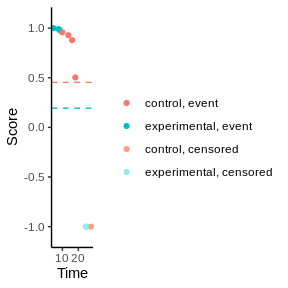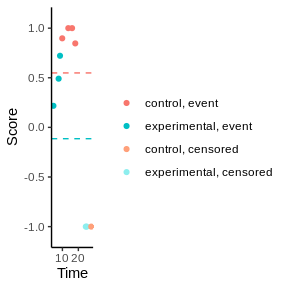# The weighted log-rank test

#### 6/23/2022

See Magirr and Burman (2019) and Magirr (2021) for details about the weighted log-rank tests, and in particular the modestly weighted log-rank test. This vignette works through an example of using the package to simulate data and perform weighted log-rank tests. A summary of the formulas used within this package is presented.

## Simulate a dataset

This package can be used to simulate a dataset for a two-arm RCT with delayed separation of survival curves by using the sim_events_delay function.

There are two parts to simulating the event times and statuses: the event model (parameters defined in event_model) and the recruitment model (parameters defined in recruitment_model).

Firstly, looking at the event model. The function sim_events_delay assumes that the survival times on the control and exponential arm follow a piecewise exponential distribution. Given rate parameter $$\lambda$$, the exponential distribution has the form:

$f(t)=\lambda \exp(-\lambda t)$

The rate parameters are set using the argument lambda_c for the control arm and lambda_e for the experimental arm. To use the piecewise version, set this argument a vector with a value for each piece. The duration of each piece is set using parameter duration_c and duration_e.

Secondly, looking at the recruitment model. The recruitment can be modeled using either a power model or a piecewise constant model. See help(sim_events_delay) more details about these models.

Additionally, the sim_events_delay function censors all observations at the calendar time max_cal_t.

Here we create a simulated dataset with 5 individuals on each arm. Assume that one unit of time is equal to one month. From entering the study until 6 months both arms have the same $$\lambda$$ parameter, with a median event time of 9 months. From 6 months, the experimental arm has a lower hazard rate, with a median event time of 18 months. Setting rec_period = 12 and rec_power = 1 means that individuals are recruited at a uniform rate over 12 months.

library(nphRCT)
set.seed(1)
sim_data <- sim_events_delay(
event_model=list(
duration_c = 36,
duration_e = c(12 ,24),
lambda_c = log(2)/9,
lambda_e = c(log(2)/9,log(2)/18)),
recruitment_model=list(
rec_model="power",
rec_period=12,
rec_power=1),
n_c=5,
n_e=5,
max_cal_t = 36
)
sim_data
#>    event_time event_status        group
#> 1       18.06            1      control
#> 2        9.89            1      control
#> 3       16.07            1      control
#> 4       28.07            0      control
#> 5       13.69            1      control
#> 6       25.22            0 experimental
#> 7       24.66            0 experimental
#> 8        8.50            1 experimental
#> 9        4.37            1 experimental
#> 10       7.64            1 experimental

## Weighted log-rank tests

Now that we have simulated a dataset, we will look at performing the weighted log-rank tests.

Consider the ordered, distinct event times $$t_1, \dots, t_k$$. Let $$d_{0,j}$$ and $$d_{1,j}$$ be the number of events at event time $$t_{j}$$ on each of the arms respectively, and let $$d_{j}$$ be equal to the sum of these two values. Similarly, let $$n_{0,j}$$ and $$n_{1,j}$$ be the number at risk at event time $$t_{j}$$ on each of the arms respectively, and let $$n_{j}$$ be equal to the sum of these two values.

The function find_at_risk can be used to calculate these values for a dataset.

find_at_risk(formula=Surv(event_time,event_status)~group,
data=sim_data,
include_cens=FALSE)
#>     t_j n_event_control n_event_experimental n_event n_risk_control
#> 1  4.37               0                    1       1              5
#> 2  7.64               0                    1       1              5
#> 3  8.50               0                    1       1              5
#> 4  9.89               1                    0       1              5
#> 5 13.69               1                    0       1              4
#> 6 16.07               1                    0       1              3
#> 7 18.06               1                    0       1              2
#>   n_risk_experimental n_risk
#> 1                   5     10
#> 2                   4      9
#> 3                   3      8
#> 4                   2      7
#> 5                   2      6
#> 6                   2      5
#> 7                   2      4

Here, each row relates to the distinct event times $$t_j$$, which are specified in column t_j. The value $$d_{0,j}$$ relates to the column n_event_control, $$d_{1,j}$$ to n_event_experimental, and $$d_{j}$$ to n_event. Similarly, $$n_{0,j}$$ relates to column n_risk_control, $$n_{1,j}$$ to n_risk_experimental, and $$n_{j}$$ to n_risk.

To calculate the test statistics for a weighted log-rank test, we need to evaluate the observed number of events on one arm, e.g. $$d_{0,j}$$, and the expected number of events on the same arm, e.g. $$d_j \frac{n_{0,j}}{n_j}$$ at each $$t_j$$. The test statistic $$U^W$$ is then a weighted sum (using weights $$w_j$$) of the difference of these values:

$U^W = \sum_{j=1}^k w_j \left(d_{0,j} - d_j \frac{n_{0,j}}{n_j}\right)$

The weights $$w_j$$ that are used depend on the type of weighted log-rank test, these are described next.

### Weights

Three types of weighted log rank test are available in this package.

• The standard log-rank test uses weights: $w_j=1$

The values of the weights in the log-rank test can be calculated using the function find_weights with argument method="lr". In the case of the standard log-rank test, the weights are clearly very simple.

find_weights(formula=Surv(event_time,event_status)~group,
data=sim_data,
method="lr",
include_cens = FALSE)
#>  1 1 1 1 1 1 1
• The Fleming-Harrington ($$\rho$$,$$\gamma$$) test uses weights: $w_j = \hat{S}(t_{j}-) ^ \rho (1 - \hat{S}(t_{j}-)) ^ \gamma$ where $$\hat{S}(t)$$ is the Kaplan Meier estimate of the survival curve in the pooled data (both treatment arms) and time $$t_j-$$ is the time just before $$t_j$$. There is the matter of choosing $$\rho$$ and $$\gamma$$. A popular choice is $$\rho=0$$ and $$\gamma=1$$ which means that the weights are equal to 1 minus the Kaplan Meier estimate of the survival curve.

Again the weights can be calculated using the find_weights function and setting method="fh", along with arguments rho and gamma.

find_weights(formula=Surv(event_time,event_status)~group,
data=sim_data,
method="fh",
rho = 0,
gamma= 1,
include_cens = FALSE)
#>  0.0 0.1 0.2 0.3 0.4 0.5 0.6
• The modestly weighted log-rank test uses weights: $w_j = 1 / \max{\{\hat{S}(t_{j}-), \hat{S}(t^\ast)\}}$ There is the matter of choosing $$t^\ast$$ or alternatively choosing the value of $$\hat{S}(t^\ast)$$. See Magirr (2021) for a discussion on this.
find_weights(formula=Surv(event_time,event_status)~group,
data=sim_data,
method="mw",
s_star = 0.5,
include_cens = FALSE)
#>  1.000000 1.111111 1.250000 1.428571 1.666667 2.000000 2.000000

### Test statistic

Under the null hypothesis that the survival curves of the two treatment arms are equal, the distribution of $$U^W$$ is

$U^W \sim N\left( 0, V^W \right)$

where the variance, $$V^W$$, is equal to $\sum_{j=1}^k w_j^2\frac{n_{0,j}n_{1,j} d_j (n_j - d_j)}{n_j^2(n_j-1)}$

The Z-statistic is then simply calculated in the usual way by dividing the test statistic $$U$$ by the square root of its variance.

To perform the full weighted log-rank test, use the function wlrt. This outputs the test statistic, its variance, the Z-statistic and the name of the treatment group the test corresponds to.

wlrt(formula=Surv(event_time,event_status)~group,
data=sim_data,
method="mw",
s_star = 0.5)
#>            u     v_u          z    trt_group
#> 1 -0.8651849 3.91482 -0.4372734 experimental

### Permutation test and scores

Leton and Zuluaga (2001) showed that every weighted log-rank test can be written as either an observed-minus-expected test (as described above), or as a permutation test.

The weights can be reformulated as scores for a permutation test using the following formula for the censoring scores and event scores respectively:

$C_j=-\sum_{i=1}w_i\frac{d_i}{n_i}$

$c_j=C_j+w_j$

These scores can be calculated using the function find_scores in the following way. Plotting these scores against the rank of the event times provides an intuitive explanation of the issues of using the Fleming-Harrington test as it makes sense that the scores for the events are decreasind with time, see Magirr (2021).

df_scores_mw<-find_scores(formula=Surv(event_time,event_status)~group,
data=sim_data,
method="mw",
s_star = 0.5)
plot(df_scores_mw)df_scores_fh<-find_scores(formula=Surv(event_time,event_status)~group,
data=sim_data,
method="fh",
rho = 0,
gamma=1)
plot(df_scores_fh)### Stratification

The issue of stratification when performing weighted log-rank tests is discussed in Magirr and Jiménez (2022). They explore various approaches to combining the results of stratified analyses. In particular they recommend combining on the Z-statistic scale, i.e. for the case of two strata, first express the stratified log-rank test as a linear combination of standardized Z-statistics, $$\sqrt{V_1}Z_1+\sqrt{V_2}Z_2 \sim N(0,V_1+V_2)$$. $$V_1$$ and $$V_2$$ are the variances for the log-rank test statistic on the first and second stratum respectively, and $$Z_1$$ and $$Z_2$$ are the Z-statistics for the log-rank test statistic on the first and second stratum respectively. Secondly, the Z-statistics $$Z_1$$ and $$Z_2$$ are replaced by the Z-statistics from the weighted log-rank test.

$\tilde{U}^W=\sqrt{V_1}\left( \frac{U_1^W}{\sqrt{V_1^W}}\right)+\sqrt{V_2}\left( \frac{U_2^W}{\sqrt{V_2^W}}\right)$

Here we introduce a strata ecog that has different $$\lambda$$ parameters, and demonstrate that it is simple to perform the described stratified weighted log-rank test.

sim_data_0 <- sim_data
sim_data_0$ecog=0 sim_data_1 <- sim_events_delay( event_model=list( duration_c = 36, duration_e = c(6,30), lambda_c = log(2)/6, lambda_e = c(log(2)/6,log(2)/12)), recruitment_model=list( rec_model="power", rec_period=12, rec_power=1), n_c=5, n_e=5, max_cal_t = 36 ) sim_data_1$ecog=1
sim_data_strata<-rbind(sim_data_0,sim_data_1)
wlrt(formula=Surv(event_time,event_status)~group+strata(ecog),
data=sim_data_strata,
method="mw",
t_star = 4
)
#> $by_strata #> strata u v_u z trt_group #> 1 ecog0 0.1615079 1.647592 0.1258256 experimental #> 2 ecog1 -2.2293871 2.386703 -1.4430662 experimental #> #>$combined
#>          u        v          z    trt_group
#> 1 -1.70296 3.316904 -0.9350569 experimental